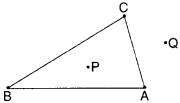NCERT Solutions for Class 6 Maths Chapter 4 Basic Geometrical Ideas Ex 4.4 are part of NCERT Solutions for Class 6 Maths. Here we have given NCERT Solutions for Class 6 Maths Chapter 4 Basic Geometrical Ideas Ex 4.4.

 Board CBSE Textbook NCERT Class Class 6 Subject Maths Chapter Chapter 4 Chapter Name Basic Geometrical Ideas Exercise Ex 4.4 Number of Questions Solved 2 Category NCERT Solutions

## NCERT Solutions for Class 6 Maths Chapter 4 Basic Geometrical Ideas Ex 4.4

Question 1.
Draw a rough sketch of a triangle ABC. Mark a point P in its interior and a point Q in its exterior. Is the point A in its exterior or in its exterior?
Solution :The point A is neither in the exterior nor in the interior of triangle ABC. It is on the triangle ABC.

Question 2.
(a) Identify three triangles in the figure.
(b) Write the names of seven angles,
(c) Write the names of the six line segments,
(d) Which two triangles have ∠B as common?
Solution :
(a) Three triangles
Triangle ABC,
Triangle ABD,$$\bar { AB }$$, $$\bar { AC }$$, $$\bar { BC }$$, $$\bar { AD }$$, $$\bar { BD }$$, $$\bar { DC }$$